## Example Questions

← Previous 1 3 4 5 6 7

### Example Question #1 : Chemistry

The significant increase in atmospheric carbon dioxide since pre-industrial levels can be seen in the world’s oceans which absorb the CO2 and in turn undergo changes in chemistry. The consequences of increased CO2 include acidification of seawater and a decrease in carbonate ion (CO32-) concentration.

Changes in seawater chemistry affect marine organisms. The early life stages of invertebrates, such as squid, may be particularly vulnerable to changes in carbon dioxide levels. Acting as both predator and prey, squid are a significant component of marine ecosystems.   For example, fish and sea birds, such as tuna and albatross, are dependent on squid as a source of prey. Furthermore, the fishing industry is impacted by the health of squid populations. California fisheries produce the majority of market squid.

In order to determine how increased levels of carbon dioxide affect the development of squid, eggs were hatched in two different conditions: normal (380 µatm) and elevated (2100 µatm) levels of CO2. The time to hatch and the size of the larval mantle (the anatomical feature that includes the body wall and fins) were measured and recorded. Two trials were conducted for each carbon dioxide concentration.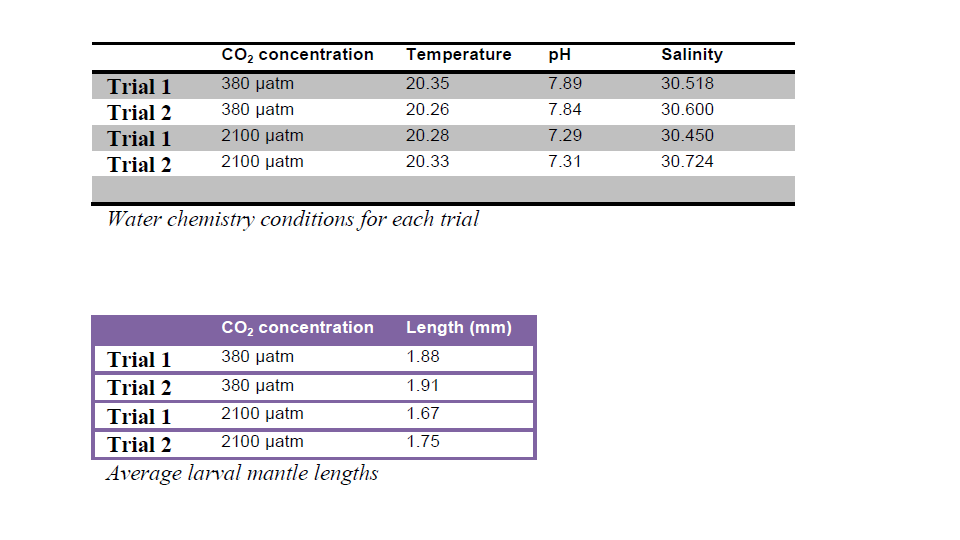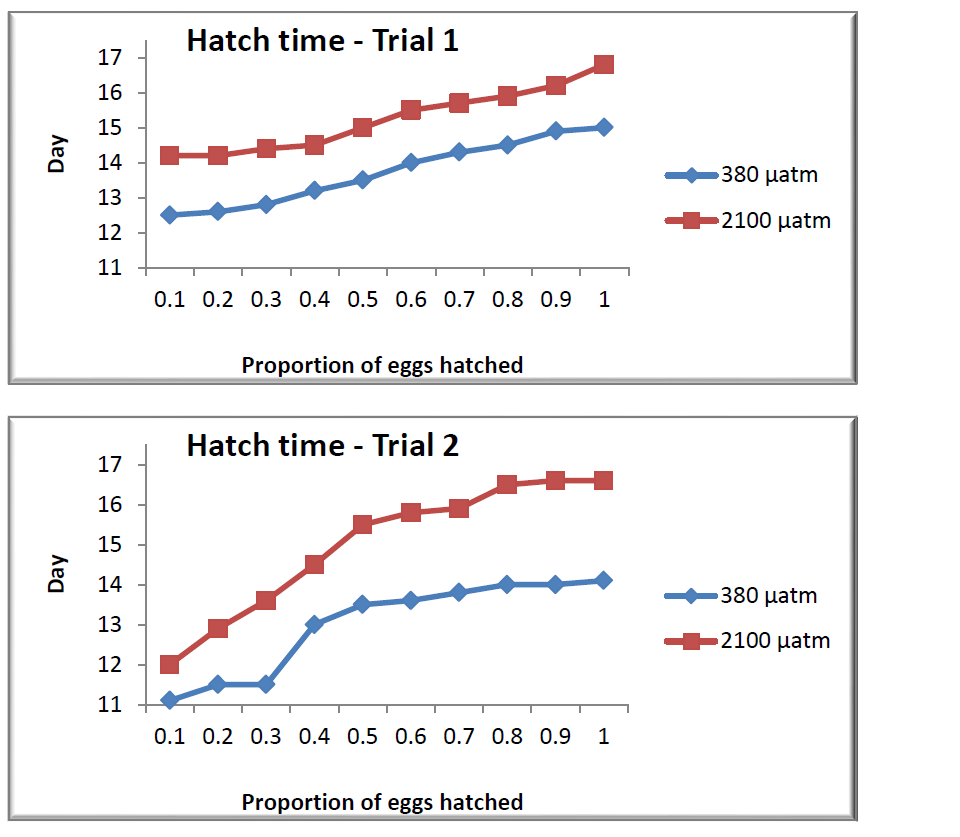Which of the following can be concluded from the passage?

Atmospheric CO2 levels correlate with the concentration of CO2 in the oceans

Tuna and albatross populations are directly related

Carbonate ion concentration correlates with ocean temperatures

Carbon dioxide concentration correlates with ocean temperatures

Atmospheric CO2 levels correlate with the concentration of CO2 in the oceans

Explanation:

Looking at the information given, the only conclusion that can be drawn is that atmospheric carbon dioxide levels correlate with ocean carbon dioxide levels.  This is evident from the first sentence of the passage:  "The significant increase in atmospheric carbon dioxide since pre-industrial levels can be seen in the world’s oceans which absorb the CO2 and in turn undergo changes in chemistry."

### Example Question #1 : How To Find Data Representation In Chemistry

A student is choosing an enzyme to use in order to complete a chemical reaction. Based on the diagram of enzyme kinetics, which enzyme should the student use?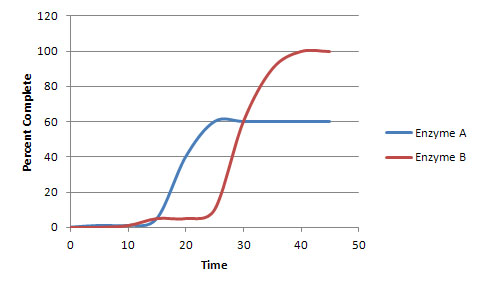Enzyme B

Both enzymes are appropriate to use

Enzyme A

Enzyme B

Explanation:

While Enzyme A has faster initial kinetics, it does not allow the reaction to reach completion. While slower, Enzyme B will allow the reaction to reach completion and should be used.

### Example Question #1 : Chemistry

A student is choosing an enzyme to use in order to complete a chemical reaction. Based on the diagram of enzyme kinetics, which enzyme is more efficient?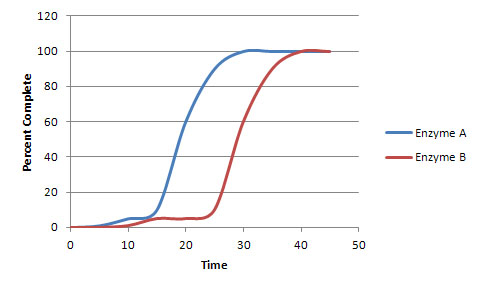Both enzymes are equally efficient

Enzyme B

Enzyme A

Enzyme A

Explanation:

In this example, both ezymes will catalyze the reaction to completion. However, Enzyme A will reach completion faster, indicating it is more efficient.

### Example Question #2 : Chemistry

The rate of a reversible chemical reaction depends on many factors, including concentrations of the reactants and products, temperature, and presence of enzymes called catalysts. In the forward reaction, two reactants combine to form one product. However, in a reverse reaction, the product is broken down into the two reactants.

In order for a forward reaction to occur, the reactants moving around in the test tube must physically interact with each other. The more often reactants interact with each other, the more produce is formed in the same amount of time. The speed at which reactants combine into products (the rate of the reaction) can be calculated by dividing the amount of a chemical produced in a reaction (often measured in moles) by the time it takes to produce that amount.

In order to determine the effects of reactant and product concentration, temperature, and presence of catalysts on the rate of a reaction, a scientist studied the following reaction: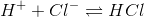The scientist varied the conditions of the experiment and measured the rate of the reaction. The results are outlined in Table 1. The units of concentration are moles per liter.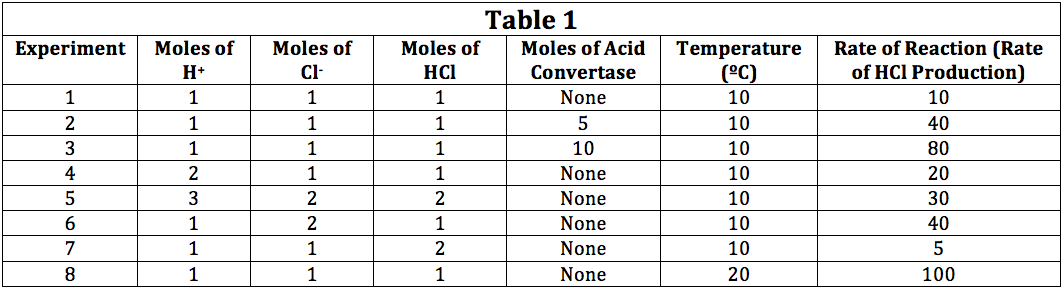If the moles of acid convertase were doubled, how would the rate of reaction change?

Remain the Same

Double

Triple

Double

Explanation:

According to Table 1, Experiments 2 and 3 show the moles of acid convertase doubling. The rate of the reaction, according to the final column, changes from 40 to 80, thus doubling.

### Example Question #1 : Chemistry

The rate of a reversible chemical reaction depends on many factors, including concentrations of the reactants and products, temperature, and presence of enzymes called catalysts. In the forward reaction, two reactants combine to form one product. However, in a reverse reaction, the product is broken down into the two reactants.

In order for a forward reaction to occur, the reactants moving around in the test tube must physically interact with each other. The more often reactants interact with each other, the more product is formed in the same amount of time. The speed at which reactants combine into products (the rate of the reaction) can be calculated by dividing the amount of a chemical produced in a reaction (often measured in moles) by the time it takes to produce that amount.

In order to determine the effects of reactant and product concentration, temperature, and presence of catalysts on the rate of a reaction, a scientist studied the following reaction:The scientist varied the conditions of the experiment and measured the rate of the reaction. The results are outlined in Table 1. The units of concentration are moles per liter.What is a possible unit of a rate of reaction?

Moles/Second

Moles/Moles

Seconds/Mole

Moles/Liter

Moles/Second

Explanation:

The passage describes that the reaction rate can be found by dividing the amount of chemicals produced in a reaction (moles) by the time it takes to produce them (seconds/minutes/hours/etc). The only answer choice that fits this pattern is moles/second. The other answer choices do contain the amount of chemicals produced but do not contain the time it takes to produce them.

### Example Question #1 : Chemistry

Both gases and liquids are considered to be fluids that have individual molecules that move around with kinetic and potential energy. Kinetic energy, defined as the energy related to motion, takes three forms: translational energy that occurs as a molecule moves from position A to position B, rotational energy that occurs as a molecule spins around an imaginary axis at its center of mass, and vibrational energy that occurs as individual atoms in a molecular bond move towards and away from each other. Usually, molecules possess varying combinations of kinetic energy forms. In contrast, potential energy is defined as stored energy that could be released to become kinetic energy. The total energy of a molecule is fixed, meaning that a molecule has some combination of kinetic and potential energies.

Varying amount of kinetic and potential energies define how molecules in a fluid interact with each other. For example, when the kinetic energy of a molecule is high (greater than 1000J), it can no longer interact with neighboring molecules strongly enough to remain a liquid. However, if the potential energies are too high (greater than 1000 J), molecules cannot escape a liquid to become a gas. If the kinetic energy is high and the potential energy is low, molecules tend to become a gas and can be modeled by an equation known as the Ideal Gas Law: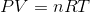Where P is the pressure of a gas, V is the volume, n is the number of moles of a gas, R is a constant, and T is temperature in degrees Kelvin.

The Ideal Gas Law perfectly applies to particles with no mass, no intermolecular interactions, and no true volume. However, real molecules do not adhere perfectly to the Ideal Gas Law.

At a constant pressure and volume, the relationship between the number of moles and temperature may best be modeled as: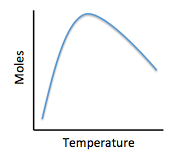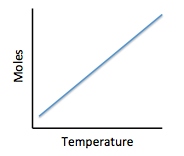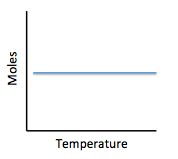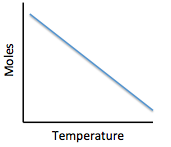Explanation:

Using the formula as a guide, we can see that number of moles and temperature are on the same side of the equals sign, indicating that they are inversly proportaion. If we rearrange the equation, we can see that: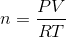. This more clearly indicates the inverse relationship. This is illustrated by a curve with a negative slope that is linear.

### Example Question #1 : Chemistry

Both gases and liquids are considered to be fluids that have individual molecules that move around with kinetic and potential energy. Kinetic energy, defined as the energy related to motion, takes three forms: translational energy that occurs as a molecule moves from position A to position B, rotational energy that occurs as a molecule spins around an imaginary axis at its center of mass, and vibrational energy that occurs as individual atoms in a molecular bond move towards and away from each other. Usually, molecules possess varying combinations of kinetic energy forms. In contrast, potential energy is defined as stored energy that could be released to become kinetic energy. The total energy of a molecule is fixed, meaning that a molecule has some combination of kinetic and potential energies.

Varying amount of kinetic and potential energies define how molecules in a fluid interact with each other. For example, when the kinetic energy of a molecule is high (greater than 1000J), it can no longer interact with neighboring molecules strongly enough to remain a liquid. However, if the potential energies are too high (greater than 1000 J), molecules cannot escape a liquid to become a gas. If the kinetic energy is high and the potential energy is low, molecules tend to become a gas and can be modeled by an equation known as the Ideal Gas Law:Where P is the pressure of a gas, V is the volume, n is the number of moles of a gas, R is a constant, and T is temperature in degrees Kelvin.

The Ideal Gas Law perfectly applies to particles with no mass, no intermolecular interactions, and no true volume. However, real molecules do not adhere perfectly to the Ideal Gas Law.

At a constant pressure, the relationship between volume and temperature may best be shown as: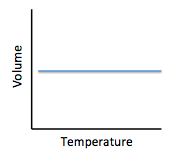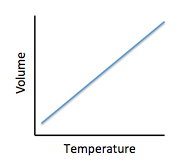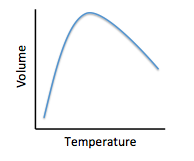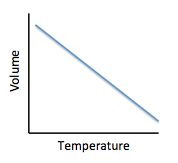Explanation:

Using the passage, we can see that, at a constant pressure, volume and temperature are both in the numerator on opposite sides of the equals sign. Thus, we can say that they are directly related to each other. The figure that best illustrates this relationship is one that is a linear, positively sloped curve.

### Example Question #1 : How To Find Data Representation In Chemistry

Chemists can model how solids, liquids, and gases behave at different temperatures and pressures with a graph called a phase diagram. When the pressure and temperature are simultaneously known, a scientist can predict whether the material will be in a specific state. The diagram is divided into sections depending on the phase and the lines between sections represent phase transitions occurring between two or more separate phases.

In general, solids of neatly stacked molecules exist when temperatures are low and pressures are intermediate. These values decrease the kinetic energy of the molecules enough to allow for attractive forces to begin the stacking process. Liquids, by contrast, are found at intermediate pressures and temperatures. The temperature is high enough to impart enough kinetic energy to prevent solid formation and the pressure is high enough to prevent the liquid from becoming a gas. Finally, a gas forms at low pressures and high temperatures. The high level of kinetic energy prevents molecules from associating with one another.

Materials can undergo processes called phase transitions, meaning they can transition from one phase to another. The transition from a solid to a liquid is called melting, while the reverse transition is called freezing. Vaporization occurs when a liquid becomes a gas, while condensation occurs when a gas becomes a liquid. Finally, in a process called sublimation, a solid can directly become a gas without passing through a liquid phase. Additionally, when a gas directly becomes a solid, this is known as deposition.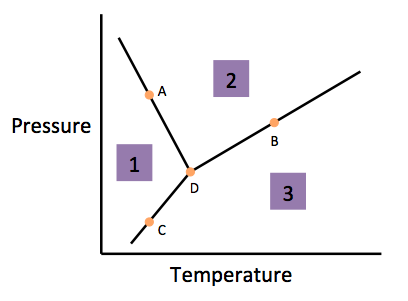According to the figure, the material represented by Area 1 is in what phase?

Solid

Gas

Cannot Be Determined

Liquid

Solid

Explanation:

According to paragraph two, solids are sets of neatly stacked molecules and exist when temperatures are low and pressures are intermediate. Looking at our figure, we can see that at low temperatures (towards the origin) and intermediate pressures, we are looking at area one. Thus, area one must be in the solid phase.

### Example Question #2 : How To Find Data Representation In Chemistry

Chemists can model how solids, liquids, and gases behave at different temperatures and pressures with a graph called a phase diagram. When the pressure and temperature are simultaneously known, a scientist can predict whether the material will be in a specific state. The diagram is divided into sections depending on the phase and the lines between sections represent phase transitions occurring between two or more separate phases.

In general, solids of neatly stacked molecules exist when temperatures are low and pressures are intermediate. These values decrease the kinetic energy of the molecules enough to allow for attractive forces to begin the stacking process. Liquids, by contrast, are found at intermediate pressures and temperatures. The temperature is high enough to impart enough kinetic energy to prevent solid formation and the pressure is high enough to prevent the liquid from becoming a gas. Finally, a gas forms at low pressures and high temperatures. The high level of kinetic energy prevents molecules from associating with one another.

Materials can undergo processes called phase transitions, meaning they can transition from one phase to another. The transition from a solid to a liquid is called melting, while the reverse transition is called freezing. Vaporization occurs when a liquid becomes a gas, while condensation occurs when a gas becomes a liquid. Finally, in a process called sublimation, a solid can directly become a gas without passing through a liquid phase. Additionally, when a gas directly becomes a solid, this is known as deposition.According to the figure, the material represented by area two is in what phase?

Gas

Solid

Liquid

Cannot Be Determined

Liquid

Explanation:

According to paragraph two, liquids are found at intermediate pressures and temperatures. The only section on the graph that corresponds to intermediate pressures and temperatures is area two.

### Example Question #1 : Chemistry

Chemists can model how solids, liquids, and gases behave at different temperatures and pressures with a graph called a phase diagram. When the pressure and temperature are simultaneously known, a scientist can predict whether the material will be in a specific state. The diagram is divided into sections depending on the phase and the lines between sections represent phase transitions occurring between two or more separate phases.

In general, solids of neatly stacked molecules exist when temperatures are low and pressures are intermediate. These values decrease the kinetic energy of the molecules enough to allow for attractive forces to begin the stacking process. Liquids, by contrast, are found at intermediate pressures and temperatures. The temperature is high enough to impart enough kinetic energy to prevent solid formation and the pressure is high enough to prevent the liquid from becoming a gas. Finally, a gas forms at low pressures and high temperatures. The high level of kinetic energy prevents molecules from associating with one another.

Materials can undergo processes called phase transitions, meaning they can transition from one phase to another. The transition from a solid to a liquid is called melting, while the reverse transition is called freezing. Vaporization occurs when a liquid becomes a gas, while condensation occurs when a gas becomes a liquid. Finally, in a process called sublimation, a solid can directly become a gas without passing through a liquid phase. Additionally, when a gas directly becomes a solid, this is known as deposition.According to the figure, the material represented by area three is in what phase?

Liquid

Solid

Gas

Cannot Be Determined